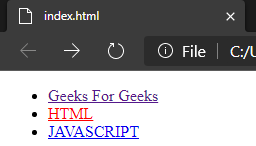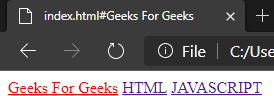# How to set active tab style with AngularJS ?

• Last Updated : 08 Sep, 2022

In this article, we will see how to set an active tab style using AngularJS, & will also understand its implementation through the example. This task can be accomplished by implementing the isActive and the ng-controller method. We will perform this task with 2 different methods.

Method 1: The ng-controller Directive in AngularJS is used to add a controller to the application. It can be used to add methods, functions, and variables that can be called on some event like click, etc to perform certain actions.

Syntax:

```<element ng-controller="expression">
Contents...
</element>```

The below example implements the above approach.

Example 1: This example describes setting the active tab style using AngularJS.

## HTML

 ```<``html``>`` ` `<``head``>``    ``<``title``>``          ``How to set active tab style with AngularJS?``      `````` ` `<``body``>``    ``<``div` `class``=``"collapse navbar-collapse"` `         ``ng-controller``=``"HeaderController"``>``        ``<``ul` `class``=``"nav navbar-nav"``>``            ``<``li` `ng-class``=``"{ active: isActive('/')}"``> ``                ``<``a` `href``=``"/"``>Geeks For Geeks ``            ````            ``<``li` `ng-class``=``"{ active: isActive('/html')}"``> ``                ``<``a` `href``=``"/html"``>HTML``            ````            ``<``li` `ng-class``=``"{ active: isActive('/js')}"``> ``                ``<``a` `href``=``"/js"``>JAVASCRIPT ``            ````        ````    ````    ``<``div` `ng-view>``    ``<``script``>``        ``function HeaderController(\$scope, \$location) {``            ``\$scope.isActive = function(viewLocation) {``                ``return viewLocation === \$location.path();``            ``};``        ``}``    ```````

Output:Method 2: Here we will use the modular function in Angular JS to create a module. A module is created by using the AngularJS function angular.module.

Syntax: for creating a module:

```<div ng-app="myFirstApp">...</div>
<script>
var app = angular.module("myFirstApp", []);

//myFirstApp refers to HTML element in which application runs.
</script>```

Syntax: for adding a directive to the module:

```<div ng-app="myApp"></div>
<script>
var my_app = angular.module("myFirstApp", []);
my_app.directive("DirectiveApp", function() {
return {
template : "Hello Geeks!!!"
};
});
</script>```

Example 2: This is another example that describes setting the active tab style using AngularJS.

## HTML

 ```<``html``>`` ` `<``head``>``    ``<``title``>How to set active tab style with AngularJS?```` ` `<``body``>``    ``<``div` `ng-app``=``"link"``> ``        ``<``a` `href``=``"#Geeks For Geeks"` `active-link``=``"active"``>``            ``Geeks For Geeks``        `` ``        ``<``a` `href``=``"#HTML"` `active-link``=``"active"``>HTML``        ``<``a` `href``=``"#JAVASCRIPT"` `active-link``=``"active"``>JAVASCRIPT ``    ````    ``<``script``>``        ``angular.module('link', []).directive('Link', ``                    ``['\$location', function(location) {``            ``return {``                ``link: function(scope, element, attrs) {``                    ``var active = attrs.activeLink;``                    ``var path = attrs.href;``                    ``path = path.substring(1);``                    ``scope.location = location;``                    ``scope.\$watch('location.path()', function(newPath) {``                        ``if(path === newPath) {``                            ``element.addClass(active);``                        ``} else {``                            ``element.removeClass(active);``                        ``}``                    ``});``                ``}``            ``};``        ``}]);``    ```````

Output:My Personal Notes arrow_drop_up